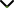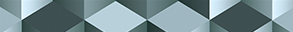# Fractional Differentiation and Integration: Above Power Law, Exponential Decay and the Generalized Mittag-Leffler Kernels

Publishing date
01 Feb 2022
Status
Closed
08 Oct 2021

Guest Editors

1King Saud University, Riyadh, Saudi Arabia

2Ambedkar University Delhi, Delhi, India

3Mugla Sıtkı Kocman University, Mugla, Turkey

This issue is now closed for submissions.

# Fractional Differentiation and Integration: Above Power Law, Exponential Decay and the Generalized Mittag-Leffler Kernels

This issue is now closed for submissions.

## DescriptionFractional calculus is a rapidly growing area of research that has attracted attention in many academic fields due to its wide applicability. In the last few decades, it has been recognized as a powerful mathematical tool to model complex real-world problems. One of the strengths of these operators is their ability to replicate processes like the power law, exponential decay memory, and even a passage from fading memory to the power law. Indeed, a number of real-world problems follow the power law, while others follow fading memory and other crossovers.

Of course, with different types of kernels, different results should be expected in theory and applications. While researchers have reached a certain level of satisfaction due to the modeling results obtained from these differential and integral operators, it is worth noting that they have not fully been able to model all real-world problems. Several novel operators have been suggested recently – for example, the concept of fractal fractional differentiation and integration, which combines fractal derivative and fractional kernels via convolution. These operators have also proven to be very efficient in modeling real-world problems with nonlocal processes and self-similar processes. However, this concept is new and needs more theoretical results and applications in different academic fields. In addition, randomness has been observed in many real-world problems and stochastic differential operators and many other methods have been adopted to solve these problems. Nevertheless, there are many physical problems following processes including non-locality and random walk. These problems cannot be modeled using neither fractional derivatives nor stochastic approaches., By combining both concepts, some of these problems could be solved with great success. There is a great need to establish new theoretical results and apply such concepts to model real-world problems arising in many fields of science and technology.

This Special Issue will therefore be devoted to collecting results in the theory and application of global differential and integral operators, piecewise differential and integral operators, and stochastic fractional differential and integral operators. Original research and review articles are welcome.

Potential topics include but are not limited to the following:

• Application of piecewise differential and integral equations to real world problems
• Application of global differentiation and integration to modeling complex problems
• Application of stochastic fractional differential and integral operators
• Theoretical results of fractional stochastic ordinary differential equations with piece-wise operators
• Theoretical results of global fractional stochastic partial differential equations
• Application of stochastic fractional differential and integral equations to chaos
• Application of piecewise differential and integral equations to epidemiology
• Piecewise fractional stochastic delay differential equations
• Delay equations with fractional global differential and integral operators
• Application of fractional stochastic partial differential equations to complex real-world problems
• Application of fractional stochastic delay partial differential and integral equations
• Piece-wise nonlinear fractional stochastic differential and integral equations with applicationsJournal metrics
See full report
Acceptance rate23%
Submission to final decision72 days
Acceptance to publication20 days
CiteScore2.600
Journal Citation Indicator1.430
Impact Factor1.9Author guidelinesEditorial boardDatabases and indexing

Article of the Year Award: Impactful research contributions of 2022, as selected by our Chief Editors. Discover the winning articles.